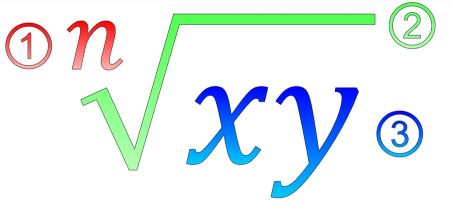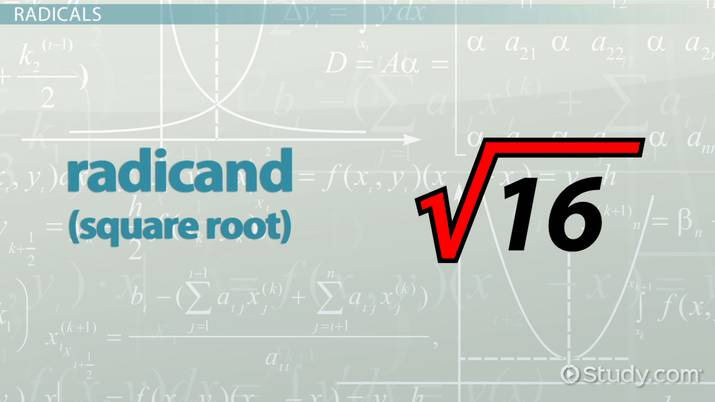# Radicand Concept in Math with Examples

Maria Airth, Jennifer Beddoe
• Author
Maria Airth

Maria has taught University level psychology and mathematics courses for over 20 years. They have a Doctorate in Education from Nova Southeastern University, a Master of Arts in Human Factors Psychology from George Mason University and a Bachelor of Arts in Psychology from Flagler College.

• Instructor
Jennifer Beddoe

Jennifer has an MS in Chemistry and a BS in Biological Sciences.

Learn about the definition of the radicand and its uses in math. Understand what a radicand symbol is. See radicand examples and know, how to solve them. Updated: 01/07/2022

Show

What is a radicand? A radicand is the object of a radical. This radicand definition might have multiple meanings because it is possible to find radicals in social sciences, math, and in political sciences. That leads directly to the next question, 'What is a radicand in math?' The radicand, in a mathematical expression, is the number found under a radical symbol.

In math, a radical symbol is used to indicate that the goal of the problem is to find the root of a number (the radicand). It is the opposite of raising numbers to a power. For example:

{eq}2 {/eq} squared is {eq}4 {/eq} and the square root of {eq}4 {/eq} is {eq}2 {/eq}.

### What Is a Radicand Symbol in Math?

The radicand symbol is commonly referred to as the radical symbol and it looks like a long division symbol with a checkmark on the front.The image above is a diagram of a radical expression. There are three parts to all radical expressions:

1. Index-The index is the portion of the expression where the intended root is indicated, such as square root, cube root, or, in this case, nth root.
3. Radicand-The radicand is the object of the problem and is the large number under the radical symbol that is being broken into its roots.

Here are a few examples of radical expressions and their meanings:

• {eq}\sqrt{4} {/eq}, this is the square root of {eq}4 {/eq}. {eq}\sqrt{4} = 2 {/eq} because {eq}2 \times 2 = 4 {/eq}
• {eq}\sqrt{27} {/eq}, this expression is seeking the cube root of {eq}27 {/eq}. {eq}\sqrt{27} = 3 {/eq} because {eq}3 \times 3 \times 3 = 27 {/eq}
• {eq}\sqrt{2000} {/eq}, finally, this expression is looking for the fifteenth root of {eq}2000 {/eq}. {eq}\sqrt{2000} = 1.66 {/eq} approximately. This is an approximate answer because the answer found on a calculator had a non-ending decimal. However, working backwards, {eq}1.66^15 = 2002.7250067 {/eq} which is very close to the desired radicand, so the rounding is a close estimate.

As you can see, the small number written high and to the left (just over the bend in the symbol) is the index and indicates what root is being sought and the radicand is the large number written under the symbol.

### History of Radicand Concept in Math

The word radicand came from the Latin word 'radix', meaning 'root' or coming from. Radix referred to roots of words as well as mathematical roots. The imagery of roots growing a tree helps to show how radicands are larger numbers grown from smaller number roots. The first usage of the word radicand in the English language was in the late 1800s, however, the current symbol for radical expressions was first developed by Rene Descartes in the 1600s. Descartes expanded on the 1500s version of a symbol being used by Christoff Rudolff to indicate taking the square root of a number. It is commonly assumed that the symbol was a form of the letter 'r' to indicate that its purpose is to find the roots of numbers (imagine today's radical symbol without the line going over the radicand).

### Radicand vs Exponent vs Index

It is easy to get confused when faced with many new terms. When working with radical expressions, there are three terms that come up frequently: radicand, exponent, and index. These are interrelated but are very different from each other. To understand the difference between these terms, refer back to the diagram of a radical expression:The number under the radical symbol from which the root is being found. It is being broken into a smaller number, which when multiplied by itself the given number of times (given by the index) will result in the original radicand (large number) again. In the diagram, the radicand is number 3.
Index
The index is the root, remember that the word radicand comes from the Latin word 'radix', meaning root. The index indicates what root to take. The most common roots are square roots (numbers that when multiplied by themselves result in the radicand) and cube roots (numbers that when multiplied by themselves three times result in the radicand). The index in the above diagram is number 1.
Exponent
Exponents are small numbers written to the top right of a number to indicate how many times a number should be multiplied by itself to reach the desired result. Exponents are the opposite of an index in a radical problem because an exponent leads to a larger number (multiplying the base by itself to reach a product) while the index in a radical seeks a smaller number than the radicand (the root from which the radicand grew).

It is easiest to understand how these terms differ when seeing them work in a problem.

• {eq}\sqrt{8} = 2 {/eq}.

Here the index is {eq}3 {/eq} indicating the desire to take the cube root (the third root) of the radicand {eq}8 {/eq}. The cube root of {eq}8 {/eq} is {eq}2 {/eq}.

• {eq}2^3 = 2 \times 2 \times 2 = 8 {/eq},An error occurred trying to load this video.

Try refreshing the page, or contact customer support.

Coming up next: What is a Quadratic Equation? - Definition & Examples

### You're on a roll. Keep up the good work!

Replay
Your next lesson will play in 10 seconds
• 1:15 History of the Radicand
• 1:42 Sample Problems
• 2:24 Real-Life Examples
• 3:29 Lesson Summary
Save Save

Want to watch this again later?

Timeline
Autoplay
Autoplay
Speed Speed

To unlock this lesson you must be a Study.com Member.

#### How do I identify the radicand?

The radicand is the number under the radical symbol (it looks like a long division symbol with a check mark at the front). If no radical symbol is used, the radicand is identified as the number whose root is being sought. For example, in the question 'What is the fourth root of 20?' the radicand is 20.

#### What is the example of radicand in math?

Radical math problems deal with finding roots of numbers (a root is a number that can be multiplied by itself to get an original number). The radicand is the original number for which a root is being sought. For example:

• What is the cube root of 8? 8 is the radicand of the question and the answer is the 2 (2 x 2 x 2 = 8)

A radicand is the number under the radical symbol. It is the number being broken into root parts. For example in the problem 'what is the square root of 4?' the radicand is 4.

### Register to view this lesson

Are you a student or a teacher?